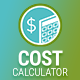How do I get 1.000,00 format for the total value?

The best way to change the total format from e.g. 1,000.00 to 1.000,00 is to modify plugin’s code.

Please locate the following line in cc.main.js in plugin’s folder. It should have the following code:

`total = total.toFixed( 2 ).replace( /(\d)(?=(\d{3})+\.)/g, '\$1,' );`

Replace the noted line with the following statements:

```var n = total, c1 = 2;
var d = ",";
var t = ".", s = total < 0 ? "-" : "";
var i = parseInt(n = Math.abs(+n || 0).toFixed(c1)) + "", j = (j = i.length) > 3 ? j % 3 : 0;

total = s + (j ? i.substr(0, j) + t : "") + i.substr(j).replace(/(\d{3})(?=\d)/g, "\$1" + t) + (c1 ? d + Math.abs(n - i).toFixed(c1).slice(2) : "");```

It should change the format of your total.

In addition, if you want to allow users to enter the numbers in text fields in the format with comma as a decimal separator, please locate the following code segment:

```window.bt_parse_float = function( x ) {
r = parseFloat( x );
if ( isNaN( r ) ) r = 0;
return r;
}```

and replace it with:

```window.bt_parse_float = function( x ) {
r = parseFloat( x.toString().replace( ',', '.' ) );
if ( isNaN( r ) ) r = 0;
return r;
}```

Please note that plugin update will override this changes. In order to save them, make sure to back up the modified file and repeat the steps from above.